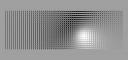# Align to Point RhinoscriptA basic Rhinoscript to rotate surfaces to align their normal to a point.

``````
Option Explicit
'Script written by Che-Wei Wang
'Script version Friday, March 07, 2008 10:55:15 AM

Call Main()
Sub Main()
'select srfs to rotate
Dim arrSurfaces:arrSurfaces=Rhino.GetObjects("select surfaces to rotate")

'select point
Dim point:point=Rhino.GetPoint("select light source point")

'calcuate vector from point to each srf
Dim srf
For Each srf In arrSurfaces
'find center of each srf
Dim arrCentroid:arrCentroid=Rhino.SurfaceAreaCentroid(srf)
'arrCentroid(0) is the center point
Dim lightVector:lightVector=Rhino.VectorCreate( point,arrCentroid(0) )

'calculate normal to srf
Dim srfNormal:srfNormal=Rhino.SurfaceNormal( srf, Array(0,0) )
'normalize vectors
lightVector=Rhino.VectorUnitize(lightVector)
srfNormal=Rhino.VectorUnitize(srfNormal)

'calcuate dot product and convert to radians
Dim Angle:Angle=acos(Rhino.VectorDotProduct( lightVector,srfNormal))

'calculate rotation axis
Dim rotAxis:rotAxis=Rhino.VectorCrossProduct(lightVector, srfNormal )

'rotate srf by that angle
Call Rhino.RotateObject(srf,arrCentroid(0),360-Rhino.toDegrees(angle),rotAxis)

Next

End Sub
``````